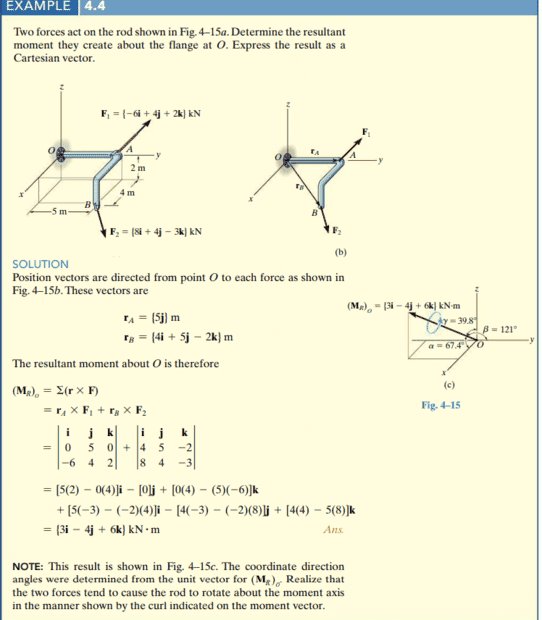# How to find the sense of rotation of a resultant moment?

• Engineering
• simphys
In summary, the sense of rotation is determined by the directions of the resultant force and the resultant perpendicular distance from that force to the axis of rotation. The moment vector is always perpendicular to the plane formed by those two vectors. To determine the sense of rotation for the resultant moment, one must calculate the resultant force and then use the right-hand rule with the displacement vector to determine the direction of rotation. This is the extra step needed when dealing with the resultant moment.f

#### simphys

Homework Statement
see picture
Relevant Equations
M = r X F
Hello, so I have a question about the sense of rotation of the body.
I get the calculating part nd stuff like that. But what I don't understand is how we would determine the sense of rotation about the moment axis?
Could someone explain this to me please? (to add to this, I know that it is determined by the right-hand rule, but how do we do that for the resultant moment?The sense of rotation is determined by the directions of the resultant force and the resultant perpendicular distance from that force to the axis of rotation.
The moment vector is always perpendicular to the plane formed by those two vectors.
http://hyperphysics.phy-astr.gsu.edu/hbase/tord.html

The sense of rotation is determined by the directions of the resultant force and the resultant perpendicular distance from that force to the axis of rotation.
The moment vector is always perpendicular to the plane formed by those two vectors.
http://hyperphysics.phy-astr.gsu.edu/hbase/tord.html
Hmm I feared that would be the case... so basically what it comes down to is that I'd need to calculate the direction angles of the resultant force, and then add the two position vector, calculate the direction angles as well and then by usnig RHR I define the sense of rotation? Or would there perhaps be a less tedious/quicker way of approaching it?

Thanks for the help

Most practical cases, in which magnitude and direction of moment or torque need to be estimated, are simpler than this problem.
On purpose, all forces and distances in this problem are off any main plane; therefore, the shown calculation process is one of the ways.
You could do the same with drawing projections, but it would not be easier.

Most practical cases, in which magnitude and direction of moment or torque need to be estimated, are simpler than this problem.
On purpose, all forces and distances in this problem are off any main plane; therefore, the shown calculation process is one of the ways.
You could do the same with drawing projections, but it would not be easier.
Thanks, but I am sorry just wanted to confirm, I mean I get that they did that on purpose as to make it a bit more complex, but what I meant was, that blue arrow. How does one calculate the sense about which it rotates about the torque axis or the way I explained in the previous comment.

Btw myapologies, perhaps you have said this and I've interpreted your comment in a different way.

Thanks, but I am sorry just wanted to confirm, I mean I get that they did that on purpose as to make it a bit more complex, but what I meant was, that blue arrow. How does one calculate the sense about which it rotates about the torque axis or the way I explained in the previous comment.

Btw myapologies, perhaps you have said this and I've interpreted your comment in a different way.
No apologies needed, my English is terrible.
I am not sure about what do you find difficult, sorry.
If the arrow is pointing towards you, the rotation is CCW; if away from you, the rotation is CW.

what I meant was, that blue arrow. How does one calculate the sense about which it rotates about the torque axis or the way I explained in the previous comment.
With your right hand, point your thumb in the direction of the arrow. Your fingers will indicate the direction of rotation.

No apologies needed, my English is terrible.
I am not sure about what do you find difficult, sorry.
If the arrow is pointing towards you, the rotation is CCW; if away from you, the rotation is CW.
it's okay, thanks. so (refer to below)
With your right hand, point your thumb in the direction of the arrow. Your fingers will indicate the direction of rotation.

So thanks guys for the help, I know that that is the direction of rotation, but..
My question is, so ofcourse, you can find the sense of rotation of ONE FORCE by the right hand rule right.
But..
When it is the RESULTANT MOMENT, to determine the sense of rotation, is this the extra step that needs to be taken?
calculating the resultant force, then the displacement vector to it and afterwards use the right hand rule to get the sense of rotation of the resultant moment?